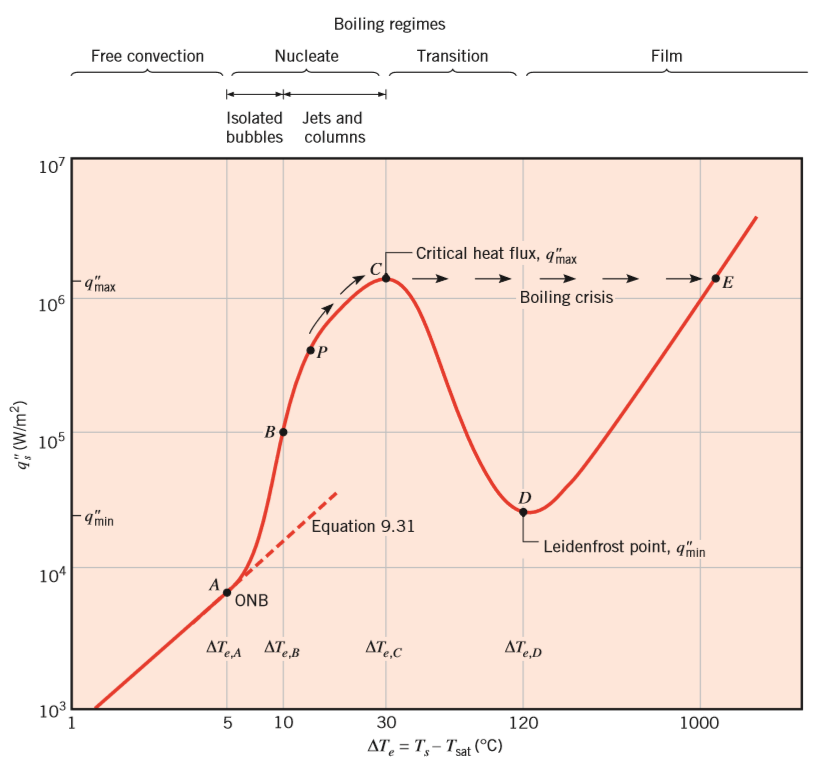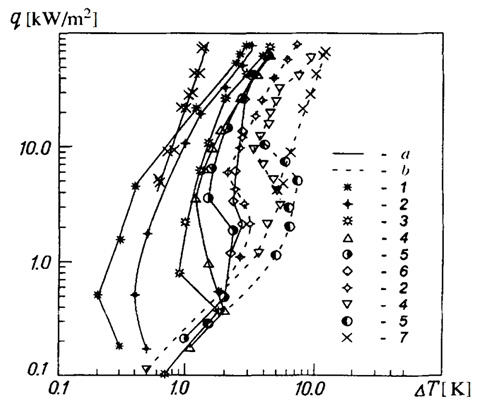# BIBLIOGAPHIC RESEARCH

A bibliographic research was done, in order to define the fundamental aspects such as the boiling regime and the critical heat flux. Once this issues were defined, a CFD code which properly represents the process was selected. This choice led to a new literature research to find a numerical model that would enable the consideration of the natural convection regime.

# Boiling regime

Nukiyama was the ﬁrst to identify different regimes of pool boiling, representing them in a curve of heat flux densities $Q$ vs the excess temperature $\Delta T_e=T{wall}-T_{sat}$. Although Nukiyama studied pool boiling of water at atmospheric pressure, similar trends characterize the behaviour of other ﬂuids. The shape of the boiling curve and its evolution under different system conditions are a result of different boiling regimes displayed in Figure 2 (Incropera et al. 2011 ).Figure 2: Typical boiling curve for water at 1 atm: surface heat ﬂux as a function of excess temperature (according to Incropera et al. 2011)

Critical heat ﬂux

The critical heat ﬂux represents an important point on the boiling curve. Kutateladze (1948)  and Zuber (1958)  obtained an expression of this value, independent of the surface material and weakly dependent of the geometry, which can be approximated as

$q_{max}=Ch_{fg}\rho_v [\frac{\sigma g(\rho_l-\rho_v)}{\rho_v^2}]^{1/4}$   (1)

For horizontal cylinders, spheres and large finite surfaces, the leading constant C is approximately equal to 0.131 (the Zuber constant). This equation applies when the characteristic length is large relative to the bubble diameter .

The value of critical heat flux of propane, under the conditions used in this project, is $q_{max}=423.32kW/m^2$ . Therefore, for the range of heat fluxes considered, the critical value is not reached and so the first two regimes can be observed: natural convection and nucleate boiling.

Pool boiling curve for propane

The pool boiling curve for propane under different pressure can be seen in the figure below (A. S. Zhuravlev, 2000 ). Curve number 5 in dashed line was considered as reference for comparison, since it represents the curve for propane in saturation conditions at P=474kPa, on a smooth surface. The free convection regime is observed in a wide range of heat flux densities, up to 5 kW/m2 and beyond this value, the nucleate boiling regime takes place, with a transition regime between 2kW/m2 and 5kW/m2.Figure 3: Curves of pool boiling of propane a) specimen with a porous coating, b) with a smooth surface;
1) P/Pcr=0.324; 2) 0.254; 3) 0.197; 4) 0.15; 5) 0.11; 6) 0.081; 7) 0.003. (Acording to A. S. Zhuravlev, 2000 )

# Boussinesq approximation

BOUSSINESQ APPROXIMATION

To represent thermal convection, the basic equations where simplified by introducing some approximations, attributed to Boussinesq (1903):

1. The variation in density, leading fluid movement, results principally from thermal effects.
2. In momentum and mass equations, density variations may be neglected except in the buoyancy force, when coupled with gravitational acceleration.

Mathematically, the Boussinesq approximation is expressed:

$\frac {\rho_l}{\rho_0} = 1- \beta(T-T_0)$  (2)

In pure natural convection, the strength of the buoyancy-induced flow is measured by the Rayleigh number:

$Ra=\frac {g\beta \Delta TL^3\rho_l}{(\mu_l \alpha')}$   (3)

where  $\beta$ and $\alpha'$ are the thermal expansion coefficient and the thermal diffusivity, defined as follow:

$\beta=- \frac{1}{\rho_l}( \frac{\partial \rho_l}{\partial T})_P$   (4)

$\alpha'=\frac {\lambda_l}{\rho_l Cp_l}$  (5)

In this study, the Rayleigh number has a value of  $Ra=6,24.10^7$ considering the highest temperature difference $\Delta T=7K$  found in literature, thus, laminar regime is expected.

The Boussinesq approximation is accurate as long as changes in temperature and therefore in actual density are small, i.e. $\beta (T-T_0)<<1$.